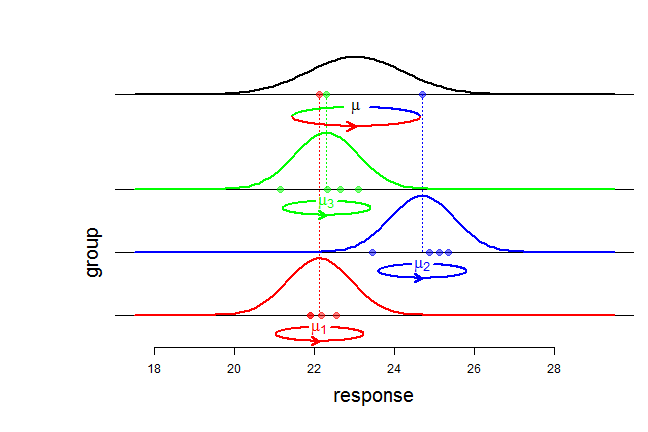1. ### The balanced ANOVA model with random effects

2014-04-06
Source

$\newcommand{\indic}{\mathbf{1}}$ $\newcommand{\perpoplus}{\overset{\perp}{\oplus}}$ $\newcommand{\RR}{\mathbb{R}}$

## The balanced ANOVA model with random effects

The balanced ANOVA model is used to model a sample $y=(y_{ij})$ with a tabular structure: $y=\begin{pmatrix} y_{11} & \ldots & y_{1J} \\ \vdots & y_{ij} & \vdots \\ y_{I1} & \ldots & y_{IJ} \end{pmatrix},$ $y_{ij}$ denoting the $j$-th response in group $i$. In the model with random effects, it is assumed that the responses $y_{ij}$ are generated in two steps. First, the group means $\mu_i$ are independently generated according to a Gaussian distribution ${\cal N}(\mu, \sigma^2_b)$ where $\mu$ is the overall mean and $\sigma^2_b$ is the so-called between variance. Second, the responses $y_{ij}$, $j =1,\ldots,J$, for each group
$i$, are independently distributed according to a Gaussian distribution ${\cal N}(\mu_i, \sigma^2_w)$ with within variance $\sigma^2_w$ and mean $\mu_i$. Shortly, the model can be written: $\begin{cases} (y_{ij} \mid \mu_i) \sim_{\text{iid}} {\cal N}(\mu_i, \sigma^2_w) & j=1,\ldots,J \\ \mu_i \sim_{\text{iid}} {\cal N}(\mu, \sigma^2_b) & i=1,\ldots,I \end{cases}.$An equivalent writing of this model, and from now on using capital letters for random variables, is $Y_{ij} = \mu + \sigma_bA_{i} + \sigma_wG_{ij},$ where all random variables $A_i$ and $G_{ij}$ are independent and $\sim {\cal N}(0,1)$.

## The three summary statistics $\bar{Y}_{\bullet\bullet}$, $SS_b(Y)$, $SS_w(Y)$

Using the tensor product language introduced in this article, the model can be written $Y = \mu({\bf 1}_I\otimes{\bf 1}_J) + \sigma_b A\otimes\indic_J +\sigma_wG, \qquad A \sim SN(\RR^I), \quad G \sim SN(\RR^I\otimes\RR^J).$

The overall mean $\bar{Y}_{\bullet\bullet}$ is given by the projection of $Y$ on the subspace $[{\bf 1}_I]\otimes[{\bf 1}_J]$:
$P_{[{\bf 1}_I]\otimes[{\bf 1}_J]} Y = \bar{Y}_{\bullet\bullet}({\bf 1}_I\otimes{\bf 1}_J).$

Then the variations $Y_{ij}-\bar{Y}_{\bullet\bullet}$ around the overall mean are given by the projection on the orthogonal complement ${\Bigl([\indic_I]\otimes[\indic_J]\Bigr)}^\perp$. Knowing that $\RR^I \otimes \RR^J = \Bigl([\indic_I]\otimes[\indic_J]\Bigr) \perpoplus \Bigl([\indic_I]^\perp\otimes[\indic_J]\Bigr) \perpoplus \Bigl([\indic_I]\otimes[\indic_J]^\perp\Bigr) \perpoplus \Bigl([\indic_I]^\perp\otimes[\indic_J]^\perp\Bigr),$ one gets \begin{align} \underset{\text{'total'}}{\underbrace{ {\Bigl([\indic_I]\otimes[\indic_J]\Bigr)}^\perp} } & = \Bigl([\indic_I]^\perp\otimes[\indic_J]\Bigr) \perpoplus \Bigl([\indic_I]\otimes[\indic_J]^\perp\Bigr) \perpoplus \Bigl([\indic_I]^\perp\otimes[\indic_J]^\perp\Bigr) \\ & = \underset{\text{'between'}}{\underbrace{\Bigl([\indic_I]^\perp\otimes[\indic_J]\Bigr)}} \perpoplus \underset{\text{'within'}}{\underbrace{\Bigl(\RR^I\otimes[\indic_J]^\perp\Bigr)}}, \end{align} thereby yielding the decomposition of the variations: $P^\perp_{[\indic_I]\otimes[\indic_J]}Y = P_{[\indic_I]^\perp\otimes[\indic_J]}Y + P_{\RR^I\otimes[\indic_J]^\perp}Y,$ whose component formulae are:

• ${\bigl(P^\perp_{[\indic_I]\otimes[\indic_J]}Y\bigr)}_{ij}=Y_{ij}-\bar{Y}_{\bullet\bullet}$

• ${\bigl(P_{[\indic_I]^\perp\otimes[\indic_J]}Y \bigr)}_{ij} = \bar{Y}_{i\bullet}-\bar{Y}_{\bullet\bullet}$

• ${\bigl(P_{\RR^I\otimes[\indic_J]^\perp}Y\bigr)}_{ij} = Y_{ij}-\bar{Y}_{i\bullet}$

Now we can see that the three summary statistics (overall mean, between sum of squares, within sum of squares) $\bar{Y}_{\bullet\bullet}, \quad SS_b(Y):={\Vert P_{[\indic_I]^\perp\otimes[\indic_J]}Y \Vert}^2, \quad SS_w(Y):={\Vert P_{\RR^I\otimes[\indic_J]^\perp}Y \Vert}^2,$ are independent random variables. Indeed, the overall mean $\bar{Y}_{\bullet\bullet}$ is given by \begin{align} P_{[{\bf 1}_I]\otimes[{\bf 1}_J]} Y &= \bar{Y}_{\bullet\bullet}({\bf 1}_I\otimes{\bf 1}_J) \\ & = \mu({\bf 1}_I\otimes{\bf 1}_J) + \sigma_b(P_{[{\bf 1}_I]}A)\otimes\indic_J+\sigma_wP_{[{\bf 1}_I]\otimes[{\bf 1}_J]}G, \end{align} the between variations are $P_{[\indic_I]^\perp\otimes[\indic_J]}Y = \sigma_b(P^\perp_{[\indic_I]} A)\otimes\indic_J + \sigma_w P_{[\indic_I]^\perp\otimes[\indic_J]} G,$ and the within variations are $P_{\RR^I\otimes[\indic_J]^\perp}Y = \sigma_wP_{\RR^I\otimes[\indic_J]^\perp} G.$ Independence follows from the independence b between $G$ and $A$ and from the orthogonality between the ranges of the different projections.

It is easy to derive $\bar{Y}_{\bullet\bullet} \sim {\cal N}\left(\mu, \frac{\sigma^2}{IJ}\right)$ with $\sigma^2=J\sigma^2_b+\sigma^2_w$. It is also easy to get $SS_w(Y) \sim \sigma^2_w\chi^2_{I(J-1)}$ because of $P_{\RR^I\otimes[\indic_J]^\perp}Y = \sigma_wP_{\RR^I\otimes[\indic_J]^\perp}G.$ To derive the law of $SS_b(Y)$, note that \begin{align} P_{[\indic_I]^\perp\otimes[\indic_J]} G & = \begin{pmatrix} \bar{G}_{1\bullet} - \bar{G}_{\bullet\bullet} & \ldots & \bar{G}_{1\bullet} - \bar{G}_{\bullet\bullet} \\ \vdots & \vdots & \vdots \\ \bar{G}_{I\bullet} - \bar{G}_{\bullet\bullet} & \ldots & \bar{G}_{I\bullet} - \bar{G}_{\bullet\bullet} \end{pmatrix} \\ & = (P^\perp_{[\indic_I]}G_{\text{row}}) \otimes \indic_J \end{align} where $G_{\text{row}} = (\bar{G}_{i\bullet})$ is the vector of row means of $G$, and then one can write
$P_{[\indic_I]^\perp\otimes[\indic_J]} Y = \bigl(P^\perp_{[\indic_I]}(\sigma_b A + \sigma_w G_{\text{row}})\bigr) \otimes \indic_J.$ Now it is easy to see that the components of $\sigma_b A + \sigma_w G_{\text{row}}$ are $\sim_{\text{iid}} {\cal N}(0, \sigma^2)$, and consequently $SS_b(Y) \sim \sigma^2\chi^2_{J-1}$.

## Confidence interval for the overall mean

By our previous derivations, the statistic $\frac{\bar Y_{\bullet\bullet} - \mu}{\frac{1}{\sqrt{I}}\sqrt{\frac{SS_b(Y)}{J(I-1)}}}$ has a Student $t$ distribution with $I-1$ degrees of freedom, wherefrom it is easy to get an exact confidence interval about the overall mean $\mu$.

Note that we would get exactly the same confidence interval if we were told only the group means $\bar{Y}_{i\bullet}$. This is the topic of the next article.